## PREDICTION_SET

Syntax

prediction_set::=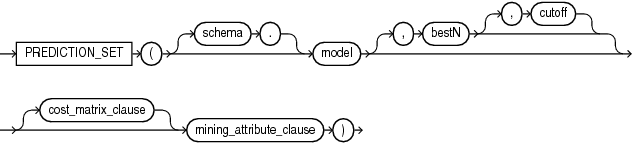Description of the illustration prediction_set.eps

Analytic Syntax

prediction_set_analytic::=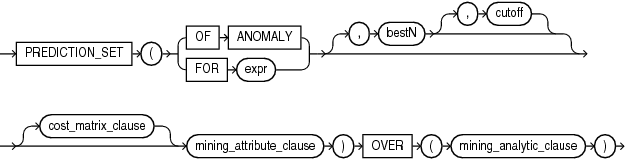Description of the illustration prediction_set_analytic.eps

cost_matrix_clause::=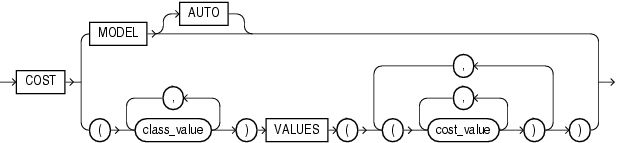Description of the illustration cost_matrix_clause.eps

mining_attribute_clause::=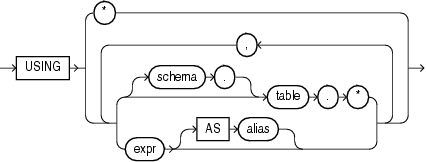Description of the illustration mining_attribute_clause.eps

mining_analytic_clause::-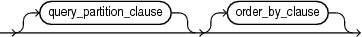Description of the illustration mining_analytic_clause.eps

for information on the syntax, semantics, and restrictions of `mining_analytic_clause`

Purpose

`PREDICTION_SET` returns a set of predictions with either probabilities or costs for each row in the selection. The return value is a varray of objects with field names `PREDICTION_ID` and `PROBABILITY` or `COST`. The prediction identifier is an Oracle `NUMBER`; the probability and cost fields are `BINARY_DOUBLE`.

`PREDICTION_SET` can perform classification or anomaly detection. For classification, the return value refers to a predicted target class. For anomaly detection, the return value refers to a classification of `1` (for typical rows) or `0` (for anomalous rows).

bestN and cutoff

You can specify `bestN` and `cutoff` to limit the number of predictions returned by the function. By default, both `bestN` and `cutoff` are null and all predictions are returned.

• `bestN` is the `N` predictions that are either the most probable or the least costly. If multiple predictions share the `N`th probability or cost, then the function chooses one of them.

• `cutoff` is a value threshold. Only predictions with probability greater than or equal to `cutoff`, or with cost less than or equal to `cutoff`, are returned. To filter by `cutoff` only, specify `NULL` for `bestN`. If the function uses a `cost_matrix_clause` with `COST MODEL AUTO`, then `cutoff` is ignored.

You can specify `bestN` with `cutoff` to return up to the `N` most probable predictions that are greater than or equal to `cutoff`. If costs are used, specify `bestN` with `cutoff` to return up to the `N` least costly predictions that are less than or equal to cutoff.

cost_matrix_clause

You can specify `cost_matrix_clause` as a biasing factor for minimizing the most harmful kinds of misclassifications. `cost_matrix_clause` behaves as described for .

Syntax Choice

`PREDICTION_SET` can score the data in one of two ways: It can apply a mining model object to the data, or it can dynamically mine the data by executing an analytic clause that builds and applies one or more transient mining models. Choose Syntax or Analytic Syntax:

• Syntax — Use the first syntax to score the data with a pre-defined model. Supply the name of a model that performs classification or anomaly detection.

• Analytic Syntax — Use the analytic syntax to score the data without a pre-defined model. The analytic syntax uses `mining_analytic_clause`, which specifies if the data should be partitioned for multiple model builds. The `mining_analytic_clause` supports a `query_partition_clause` and an `order_by_clause`. (See .)

• For classification, specify `FOR` `expr`, where `expr` is an expression that identifies a target column that has a character data type.

• For anomaly detection, specify the keywords `OF ANOMALY`.

The syntax of the `PREDICTION_SET` function can use an optional `GROUPING` hint when scoring a partitioned model. See GROUPING Hint.

mining_attribute_clause

`mining_attribute_clause` identifies the column attributes to use as predictors for scoring. When the function is invoked with the analytic syntax, these predictors are also used for building the transient models. The `mining_attribute_clause` behaves as described for the `PREDICTION` function. (See .)

Note:

The following example is excerpted from the Data Mining sample programs. For more information about the sample programs, see Appendix A in Oracle Data Mining User's Guide.

Example

This example lists the probability and cost that customers with ID less than 100006 will use an affinity card. This example has a binary target, but such a query is also useful for multiclass classification such as low, medium, and high.

```SELECT T.cust_id, S.prediction, S.probability, S.cost
FROM (SELECT cust_id,
PREDICTION_SET(dt_sh_clas_sample COST MODEL USING *) pset
FROM mining_data_apply_v
WHERE cust_id < 100006) T,
TABLE(T.pset) S
ORDER BY cust_id, S.prediction;

CUST_ID PREDICTION  PROBABILITY         COST
---------- ---------- ------------ ------------
100001          0   .966183575   .270531401
100001          1   .033816425   .966183575
100002          0   .740384615  2.076923077
100002          1   .259615385   .740384615
100003          0   .909090909   .727272727
100003          1   .090909091   .909090909
100004          0   .909090909   .727272727
100004          1   .090909091   .909090909
100005          0   .272357724  5.821138211
100005          1   .727642276   .272357724
```